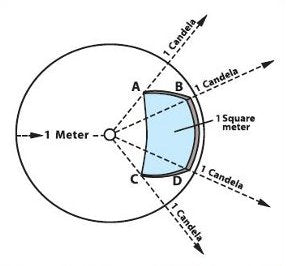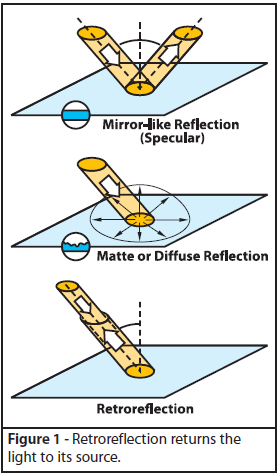# Retroreflection Measurement##### It seems intuitively correct to measure retroreflection as a ratio of the intensity of light returned in the direction of the driver to the intensity of their car's headlights. This ratio would give a scale for retroreflection that consisted of a similitude (dimensionless number) between 0 and 1. Unfortunately there are practical problems with this approach. In addition, there must be a system of units to define light flux, intensity and other optical quantities.##### In Figure 6, the solid angle subtended by the Area "ABCD" is equal to the area of "ABCD" divided by the total area of the concentric sphere multiplied by the total number of steradians in the sphere. The equation looks like:##### With the understanding of solid angles, the definition of optical quantities can be made. The basic optical quantity is the 'candela', which is a measure of luminous intensity. The candela is the luminous intensity of a source emitting a monochromatic radiation in a given direction of frequency 540 x 10¹² Hertz or wavelength 555 nanometers. The radiant intensity of which, in that direction, is 1/683 watts per steradian. This definition, while not helpful for an intuitive grasp of the nature of luminous intensity, does give us a physical means to establish optical units.